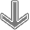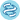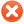More optionsShow cutoff value used in analysis: Show probe expression range: Generate high resolution TIFF file: Invert HR values below 1: Export plot data as text: Compute median over entire dataset:Backward reference:Use earlier release of the database: all 2010 (n=1809) 2012 (n=2960) 2014 (n=4142) 2017 (n=5143)Quality controlRemove redundant samples: (n=1293)*Use array quality control: *exclude biased arrays (n=5136): exclude outlier arrays (n=4665): do not filter for array quality (n=5143): Check proportional hazards assumption:*force proportionality assumption:Perform multivariate analysis for (n=5143): MKI67: ESR1: HER2 (ERBB2): *Turning off this feature will add a watermark to the KMplot.
 Remember filtering settings:
 Affy id/Gene symbol:Split patients by --none--lower quartilelower tertilemedianupper tertileupper quartile Auto select best cutoffTrichotomization: --none--T1 vs T3Q1 vs Q4Survival: RFS (n=3955)OS (n=1402)DMFS (n=1747)PPS (n=414)Compute median survival:Follow up threshold: all2 months4 months6 months12 months18 months24 months30 months36 months48 months60 months120 months180 months240 monthsCensore at threshold:## Probe set options

Use
 user selected probe set all probe sets per geneonly JetSet best probe setPlot beeswarm graph of probe distribution:Show probe expression in normal tissue:## Restrict analysis to subtypes...

 ER status: (n=3779) all ER positive (n=2565) ER negative (n=1214) derive ER status from gene expression data: (n=5143)PR status: (n=1982) all PR positive (n=954) PR negative (n=1028) HER2 status: (n=1872) all HER2 positive (n=416) HER2 negative (n=1456) Intrinsic subtype: (n=5143)all basal (n=879) luminal A (n=2504) luminal B (n=1425) HER2+ (n=335)Lymph node status: (n=3720) all Lymph node positive (n=1459) Lymph node negative (n=2259) Grade: (n=2545) all 1 (n=378) 2 (n=1077) 3 (n=1090) TP53 status: (n=595)all mutated (n=232) wild type (n=363) Pietenpol subtype: (n=1246) all basal-like 1 (n=239) basal-like 2 (n=97) immunomodulatory (n=290) mesenchymal (n=229) mesenchymal stem–like (n=115) luminal androgen receptor (n=276)

## Restrict analysis to selected cohorts...

 systemically untreated patients: include (n=1015)exclude (n=2884) patients with following systemic treatment: endocrine therapy:any (n=3099)include (n=1381)exclude (n=1718)tamoxifen only (n=1043) chemotherapy: any (n=3654)include all (n=1543)adjuvant only (n=678)neoadjuvant only (n=884)exclude all (n=2105) patient cohort similar to SEER prevalencesUse following dataset for the analysis: alleach separatelyE-MTAB-365 (n=537)E-TABM-43 (n=37)GSE11121 (n=200)GSE12093 (n=136)GSE12276 (n=204)GSE1456 (n=159)GSE16391 (n=55)GSE16446 (n=120)GSE16716 (n=47)GSE17705 (n=196)GSE17907 (n=54)GSE18728 (n=61)GSE19615 (n=115)GSE20194 (n=45)GSE20271 (n=96)GSE2034 (n=286)GSE20685 (n=327)GSE20711 (n=90)GSE21653 (n=240)GSE2603 (n=99)GSE26971 (n=276)GSE2990 (n=102)GSE31448 (n=71)GSE31519 (n=67)GSE32646 (n=115)GSE3494 (n=251)GSE37946 (n=41)GSE41998 (n=279)GSE42568 (n=121)GSE45255 (n=139)GSE4611 (n=153)GSE5327 (n=58)GSE6532 (n=82)GSE7390 (n=198)GSE9195 (n=77)

Please note: the generated p value does not include correction for multiple hypothesis testing by default.n = number of patients with available clinical data

Please kindly cite our paper to support further development: Gyorffy B, Lanczky A, Eklund AC, Denkert C, Budczies J, Li Q, Szallasi Z. An online survival analysis tool to rapidly assess the effect of 22,277 genes on breast cancer prognosis using microarray data of 1809 patients, Breast Cancer Res Treatment, 2010 Oct;123(3):725-31.# Enter more genes## Genes selected:

 Use mean expression of the selected probes:

## Paste list of probeset IDs

HGU133A and HGU133Aplus2 probe set IDs only. Each new ID must be entered into a new line. Maximum 65 genes are allowed.

KMplot.com Copyright © 2009-2019 | Contact